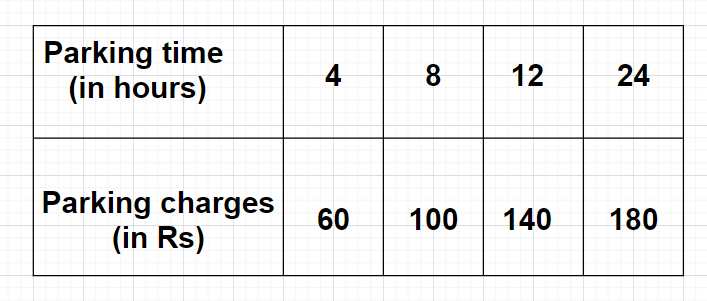Courses
Courses for Kids
Free study material
Free LIVE classes
MoreLIVE
Join Vedantu’s FREE Mastercalss

# The below table gives the data for car parking charges near a railway station up to the corresponding parking time.Check if the parking charges are in direct proportion to the parking time.Verified
363.6k+ views
Hint: Here, we will proceed by finding out all the ratios of the parking charges to the parking time obtained with the help of the given data and then comparing these ratios. If these ratios are equal to each other then only we will be saying that the parking charges are in direct proportion to the parking time.

Corresponding to parking time 4 hours and parking charges Rs 60, the ratio of parking charges to the parking time (in Rs per hour) is $\dfrac{{60}}{4} = \dfrac{{15}}{1} = 15:1$
Similarly, corresponding to parking time 8 hours and parking charges Rs 100, the ratio of parking charges to the parking time (in Rs per hour) is $\dfrac{{100}}{8} = \dfrac{{25}}{2} = 25:2$
Similarly, corresponding to parking time 12 hours and parking charges Rs 140, the ratio of parking charges to the parking time (in Rs per hour) is $\dfrac{{140}}{{12}} = \dfrac{{35}}{3} = 35:3$
Similarly, corresponding to parking time 24 hours and parking charges Rs 180, the ratio of parking charges to the parking time (in Rs per hour) is $\dfrac{{180}}{{24}} = \dfrac{{15}}{2} = 15:1$
Note: Two variables (let it be x and y) are said to be in direct proportion to each other when $x \propto y \Rightarrow x = ky \Rightarrow \dfrac{x}{y} = k$ where k is any constant. This means that any two variables like x and y are in direct proportion to each other only when their ratio is constant or different values of variables x and y always obtain the same ratio.